﻿ MATS347 Composites Design and Manufacture
 Composites Design and Manufacture (Plymouth University teaching support materials)Anisotropic elasticity theory. LecturePowerPoint ReadingLists Reviewpapers SubjectIndexAnisotropic materials possess properties which are dependent on the direction in the material.  In order to present a generalised Hooke's Law for the various possibilities it will be assumed that the material is homogeneous.  In this case, the stress and strain will be specified by second-rank tensors.  All components of the strain will be linearly related to all the components of the applied stresses.  Nine stress terms will exist: σxx, σyy, σzz, σxy, σyx, σxz, σzx, σyz and σzy.  If the x, y and z directions are referred to in terms of the integers 1, 2, 3, then the generalised Hooke's law may be written: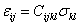where i, j, k, l may each be any of the integers from 1 to 3.  Cijkl form a fourth-rank tensor and are termed the material compliances and εij is a second-rank mathematical strain tensor.  Similarly, the stress-strain tensor is given by: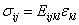where Eijkl are referred to as the material stiffness or the terms of the modulus tensor.

Cijkl contains 81 terms (34) and from the definitions of the components of the stress and strain tensors, the number of independent terms reduces to 36 because: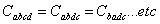The finite element method is based on matrix algebra and it is therefore necessary to convert Cijkl from tensor to matrix form.  The relationship between stress and strain tensors can be defined using matrices, with the four tensor suffices reduced to two matrix suffices according to:

 Tensor: 11 22 33 12 13 21 23 31 32 Matrix: 1 2 3 6 5 6 4 5 4
Factors of 2 are introduced to cater for differences between tensorial and engineering strains:
• Cijkl = Cmn when both m and n lie between 1 and 3
• 2Cijkl = Cmn when either m or n lie between 4 and 6
• 4Cijkl = Cmn when both m and n lie between 4 and 6

As an example, the matrix component C45 will be equivalent to tensor component 4C2331.  The tensor equations may this be contracted to matrix and vector operations, in engineering rather than in mathematical strains: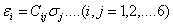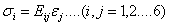The Cij matrix still contains 36 terms, but due to symmetry (represented by sym at lower left in the equations below) only 21 are independent, and because of Maxwell's reciprocal theorem this number further reduces to nine independent terms.

Composite materials, such as fibre reinforced plastics are often referred to as anisotropic.  In practice, these materials usually exhibit planes of symmetry with the principal constants not dependent on position, and are more correctly termed "orthotropic".  Normally the Cij matrix is referred to the planes of material symmetry so that the components of the matrix are aligned with the principal directions of the material (the material symmetry axes xm, ym and zm are also defined as the 1,2,3 axes).  A generally orthotropic material has three planes of symmetry and the compliance matrix reduces to nine independent constants:The zero terms occur when the reference axes coincide with the material symmetry axes, and may otherwise be non-zero due to shear coupling effects.  Simple relationships exist between the components of the [Cm] and the elastic moduli of the material:where
• E and G are the Young's modulus and shear modulus respectively
• 11, 22 and 33 refer to the xm, ym and zm directions
• 12, 13 and 23 are associated with the (xm-ym),  (xm-zm) and (ym-zm) planes
• ν12 etc are the Poisson's ratio values.

A transversely isotropic material is isotropic about one axis. For example, a unidirectional composite has fibres running parallel to the xm axis (the 1-axis).  This additional symmetry causes a reduction in the number of terms in the compliance matrix because: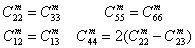A square symmetric material is isotropic in one plane because there are equal amounts of reinforcement in two mutually perpendicular directions (as in a bidirectional laminate).  If the reinforcement is in the zm plane (the 3-plane), then: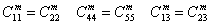The compliance matrix retains the nine terms, but only six of these are now independent.

The formulation of the expressions for the element stiffness matrix of two-dimensional in-plane finite elements is presented elsewhere (webpage yet to be constructed).  The only matrix affected by the introduction of material anisotropy is the stress-strain matrix [D].  This matrix for an orthotropic material is set up by calculating the elements of the compliance matrix [Cm] since these are easily obtained from the relevant material moduli and Poisson's ratios.  [Cm] is then inverted to give the stress-strain matrix [Dm] corresponding to [D] for the isotropic case.  It is usual to relate the material xm, ym planes to the global (structural) xg, yg plane so that the xm, ym axes lie in the xg, yg plane.

The stress-strain matrix in the finite element stiffness expression is related to the stresses and strains along the element axes (xe, ye, ze).  When the material symmetry axes coincide with the element axes, the stress-strain equations in matrix form are:If the element axes are oriented at an angle θ to the material symmetry axes, then the stress-strain matrix must be transformed to give the modified compliances associated with the element axes.  The stress-strain equations will also need to be modified if the material ym-zm axes are interchanged with the structural yg-zg axes so that the ym axis now coincides with the global xg-yg plane.  Simplifications arise when the finite element analysis of a composite material is limited to two dimensional (plane stress or plane strain) models.  The general cases in which the element axes (xe, ye) are not coincident with the material symmetry axes (xm, ym), although they must be in the same plane, will be considered.

Plane stress

In this case, several stresses are interdependent: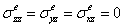As only two-dimensions are being considered, the stress-strain relationship simplifies to:and the inverse becomes:where the subscript, σ, indicates plane stress, and where σDije is the reduced stress-strain matrix: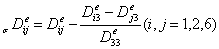The elements of the stress-strain matrix are now referred to as σD rather than E.  This is because the 3x3 compliance matrix has been inverted rather than the full 6x6 matrix.  Plane stress analysis requires only the compliances C11m, C12m,C22m and C66m.

Plane strain

By definition, εxxe = εxye = εxze = 0 and σyze = σxze = 0.  The stress-strain relationship reduces to:and the inverse:where the subscript ε indicates plane strain and where εCije are the reduced compliances in the element axes: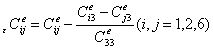The D elements of the stress-strain matrix are exactly the same as those from the full 6x6 matrix (i.e. the inverse of [Cm]).  To obtain them it is necessary to know seven elements of the compliance matrix: C11m, C12m, C13m, C22m, C23m, C33m, C66m.

Reference

The above text is taken directly from J Summerscales, The Mechanical Properties of Carbon Fibre with Glass Fibre Hybrid Reinforced Plastics, PhD thesis, CNAA/Plymouth Polytechnic, May 1983 and is derived from The PAFEC 75 Handbook: Theory - Results, PAFEC Limited, Nottingham, December 1975.